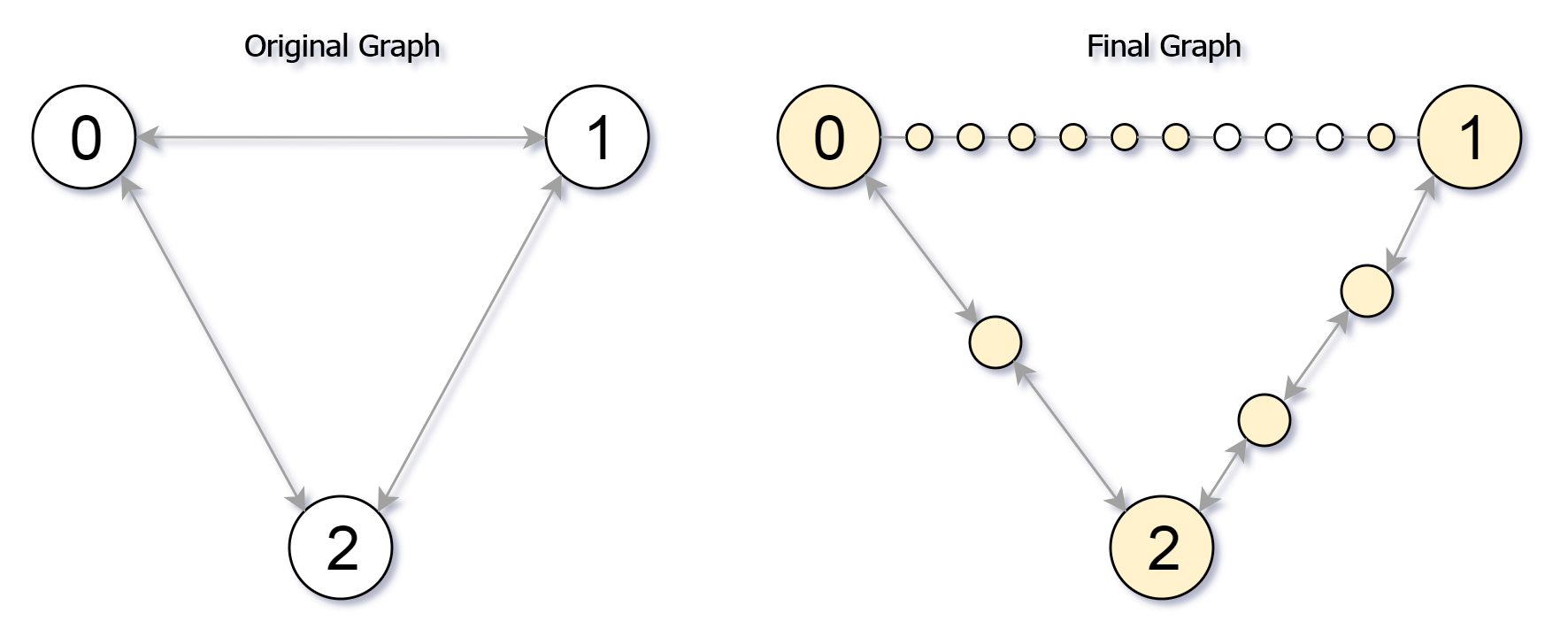# 882.reachable nodes in subdivided graph## Description

Starting with an undirected graph (the “original graph”) with nodes from 0 to N-1, subdivisions are made to some of the edges.

The graph is given as follows: edges[k] is a list of integer pairs (i, j, n) such that (i, j) is an edge of the original graph,

and n is the total number of new nodes on that edge.

Then, the edge (i, j) is deleted from the original graph, n new nodes (x_1, x_2, …, x_n) are added to the original graph,

and n+1 new edges (i, x_1), (x_1, x_2), (x_2, x_3), …, (x_{n-1}, x_n), (x_n, j) are added to the original graph.

Now, you start at node 0 from the original graph, and in each move, you travel along one edge.

Return how many nodes you can reach in at most M moves.

### Example 1:

``````Input: edges = [[0,1,10],[0,2,1],[1,2,2]], M = 6, N = 3
Output: 13
Explanation:
The nodes that are reachable in the final graph after M = 6 moves are indicated below.
``````### Example 2:

``````Input: edges = [[0,1,4],[1,2,6],[0,2,8],[1,3,1]], M = 10, N = 4
Output: 23
``````

### Note:

• 0 <= edges.length <= 10000
• 0 <= edges[i] < edges[i] < N
• There does not exist any i != j for which edges[i] == edges[j] and edges[i] == edges[j].
• The original graph has no parallel edges.
• 0 <= edges[i] <= 10000
• 0 <= M <= 10^9
• 1 <= N <= 3000
• A reachable node is a node that can be travelled to using at most M moves starting from node 0.

1. updating

## 参考代码

``````updating
``````